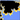# Mandelbrot Set

17 March 2009 — written by Prakash PrasadThe Mandelbrot set was named after the mathematician Benoit Mandelbrot. The Mandelbrot set is one of the most famous examples of a fractal and the process of generating it is based on an extremely simple equation involving complex numbers.

The Mandelbrot set is a set of complex numbers, so we graph it on the complex number plane. However, first we have to find many numbers that are part of the set. To do this we need a test that will determine if a given number is inside the set or outside the set. The test is based on the equation:

$Z = {Z}^2 + C$

$C$ represents a constant number, meaning that it does not change during the testing process. $C$ is the number we are testing; the Point on the complex plane that will be plotted when testing is complete.

$Z$ starts out as zero, but it changes as we repeatedly iterate this equation. With each iteration we create a new $Z$ that is equal to the old ${Z}^2$ plus constant $C$. So the number $Z$ keeps changing throughout the test.

As we iterate our equation, $Z$ changes and the magnitude of $Z$ also changes. The magnitude of $Z$ will do one of two things. It will either stay equal to or below 2 forever, or it will eventually surpass two. Once the magnitude of $Z$ surpasses 2, it will increase forever. In the first case, where the magnitude of $Z$ stays small, the number we are testing is part of the Mandelbrot set. If the magnitude of $Z$ eventually surpasses 2, the number is not part of the Mandelbrot set. As we test many complex numbers we can graph the ones that are part of the Mandelbrot set on the complex number plane.